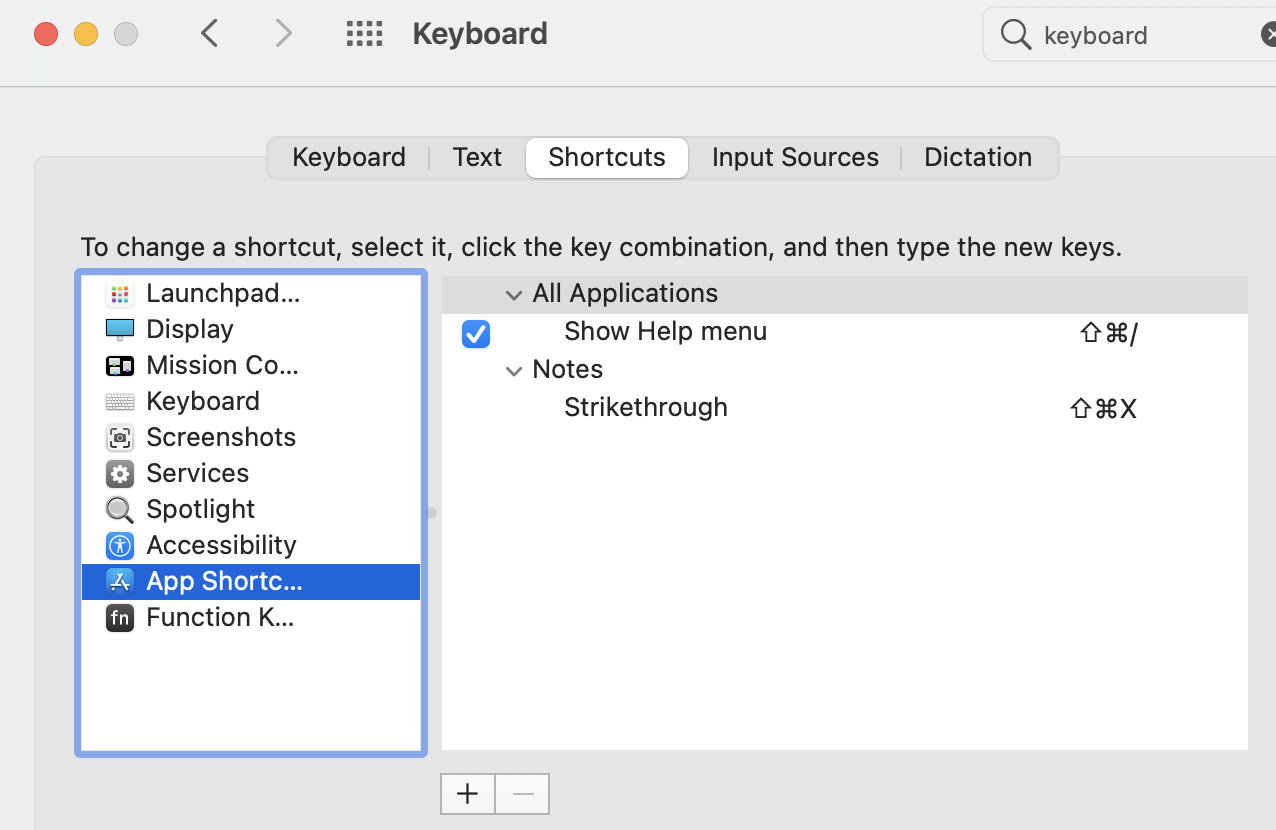### Practical Text Manipulation using MID LEFT RIGHT FIND Functions in MS Excel

Text manipulation is one of the great features of MS Excel. It has a lot of built-in functions to extract desired values from a text. By using different combinations of these functions we can create a complex formula.

I have listed a few practical examples which are very useful.

Extracting a Door number from an address text.

 8314 W. Galvin Lane West Des Moines, IA 50265 68 4th Ave. Neptune, NJ 07753 8 Oklahoma St. Ladson, SC 29456

I will use the LEFT and FIND functions to extract the Door number of an address.

=LEFT(B4,FIND(" ",B4))

Here I am using the delimiter space (" "). The formula first finds a space character which appears after the door number. Then extracts the value whatever if at the left side of the first space.

Extracting a ZIP code from an address

Generally, the ZIP code will at the end of the address. Using the RIGHT function, we can extract the ZIP code

=RIGHT(B9,5)

Extracting a specific number from anywhere in the text

Suppose, from the below text I need to extract the dollar amount Which may be present anywhere in the text. For this, I will use multiple functions. For better understanding and for clear visibility,  I have separated the functions. If you understand them clearly then you can create your own complex formula.

I had \$100 in my pocket
bought 2 pens for \$1 each
Spent \$50 for snacks.
Now I have \$48 left

First, identify the starting position of the Dollar symbol.

 =FIND("\$",B10) Then identify the next
Then identify the next space after the Dollar symbol and the digits using the below functions

=FIND(" ",B10,FIND("\$",B10))

Finally, extract the context between the first index, tha is a dollar sign and the space character after the amount. For this, I am using the mid function

=MID(B10,C10,D10-C10)

Extracting the names from a text

The below data has names mixed with some other text. The left part does not have any unwanted text. So we don't have to worry about the left part. But the right portion has other text and we want to remove that. The text marked in red are to be removed.

John Smit (IT Dept)
Krishh J (Sales)
Priore Priore (Software)

Lucy S (Sales)

It is very clear that we need to extract the text up to the first "(" symbol. This is can be done using a FIND and MID functions. Note the -1 at the end of the formula. This is to exclude the "(" character. Because the FIND function will return the position of the "(". But we don't need it.

=LEFT(B17,FIND("(",B17)-1)

1.2.3.4.5.6.7.8.### Apple MacBook Notes strike through Menu option and Setting Keyboard shortcut.

Apple Notes is the probably most widely used App for taking notes on Apple MacBook or Apple Mac in general. In this tutorial, I will show y...